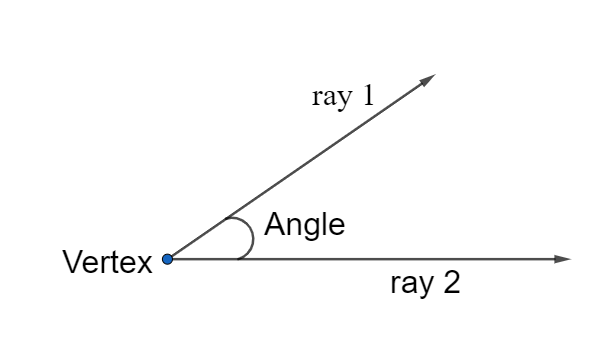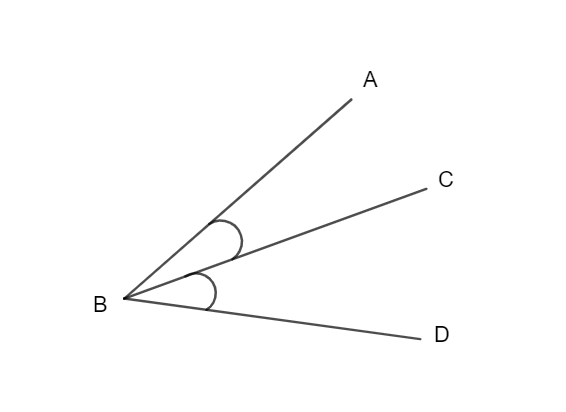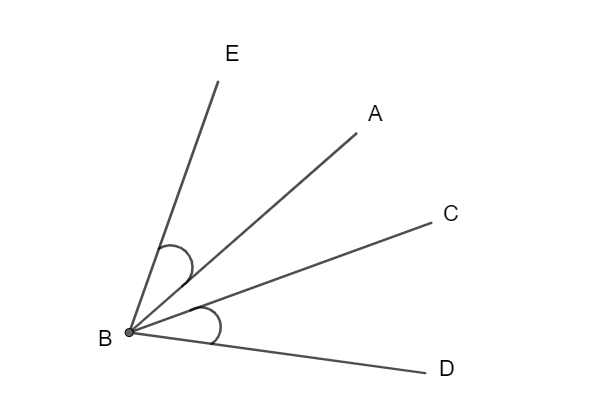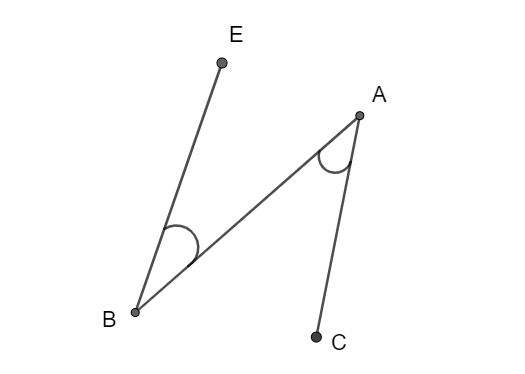In math, adjacent angles are an important topic to understand. Adjacent angles share a common side and a common vertex, but they do not cross in any way. When you break down the phrase adjacent angles, you can see exactly what it means. It is two angles that are adjacent to one another. It’s important to note that adjacent angles must have the same side and vertex. As a result, if two angles come from the same corner but are divided by another angle in the middle, they do not share any sides. Because they don’t share a side AND a vertex, they aren’t neighboring angles.It means two angles that are adjacent to one another. Though the angels have a common side and vertex, they don’t overlap in any way.

Adjacent angles refer to two angles that share the same vertex and side.

However, before we can define adjacent angles, we must first determine what angle is. In a geometric plane, an angle is a figure formed by combining two rays that share a common endpoint. The sides of the angle are called the rays, and the common endpoint is termed the angle’s vertex.You can see how we can generate an angle by combining two rays in the diagram above. We can consider the two rays, ray 1 and ray 2, to be an arm of the angle. Now, the common point is said to be the angle’s vertex, as described in the definition.

We can also generate angles by the crossing of two planes. Two curves in a plane and a variety of other methods can also work.

Let’s have a look at the definition of adjacent angles now.

### Definition

When two angles share a common side with a common point without overlapping are called adjacent angles.Angle ABC and CBD are adjacent angles in the diagram above.

Three reasons that made them A. Angle is,

1.  As stated in the definition of neighboring angle, they have a common line or common side CB.
2. According to the definition of neighboring angles, they have a common point or common vertex B.
3. As stated in the definition, the angles do not overlap.

Let’s look at some adjacent angle samples.Because they share a common vertex but not a common side, the angles EBA and CBD in the diagram above are not adjacent. As a result, these two angles are not adjacent.The angles EBA and BAC in the diagram above are not adjacent. It is because they share a shared side but not a common vertex. As a result, these two angles are not adjacent.

Here’s a quick rundown of the properties of adjacent angles to help you picture what they look like.

1. They have a common side.
2. They have a vertex in common.
3. The angles do not cross one other.
4. Although they share a common side in the middle, they do not share the other side.
5. They don’t have a common point on the inside.
6. They might be either complementary or additional.

The simplest way to recognize an adjacent angle is to identify a common side and a common vertex. When two angles have the same side and come from the same corner, they are called adjacent angles.

It’s important to note that adjacent angles must have the same side and vertex. For example, two angles that come from the same corner do not share any sides. They are separated by another angle in the middle. Because they don’t share a side AND a vertex, they aren’t adjacent angles.

Practice makes it simpler to recognize adjacent angles. Although seeing examples will help you understand what you’re searching for.

### Adjacent angle vs vertical angle

In geometry, being able to compare neighboring and vertical angles is a vital ability to master. Imagine two straight lines meeting to form a cross to better understand the difference between these two types of angles.

Four angles are produced when a cross is made. Because they have a common side and a common vertex, we may identify nearby angles. However, how can we determine a vertical angle? It’s just as simple to locate a vertical angle as it is to find an adjacent angle. A group of vertical angles, like adjacent angles, will have the same vertex position. However, they do not have to be on the same side.

When considering a cross, the vertical angles are those that are straight to each other. It’s for this reason that they’re sometimes referred to as vertically opposite angles.

### Adjacent angle in a parallelogram

In a parallelogram, adjacent angles are two angles on the same side of the parallelogram.

When the sum of these two angles is 90 degrees, the lines form complementary angles. On the other hand, two lines form supplementary angles when the sum is 180 degrees.

If two quadrilateral angles share a common side as an arm, they are called adjacent angles.

Two neighboring supplementary angles that share a side and vertex and add up to 180 degrees are known as adjacent supplementary angles.

Look at the picture below to see which angles are nearby supplemental using this concept.Because line segment AB and vertex B are shared by angles ABC and ABD, they are adjacent.

Because they have no common side, the angles EFG and HIJ are not contiguous.

However, both pairs of supplementary angles (ABC and ABD, and EFG and HIJ) have angle measurements of 180 degrees.

To summarise, EFG and HIJ are complementary angles. However, ABC and ABD are adjacent supplementary angles.

1. Q. What are the pairs of adjacent angles in the following image?Ans:

First of all ∠ APD and ∠ DPC are adjacent angles.

∠ DPC and ∠ CPB are also adjacent to each other.

Similarly, ∠ APD and ∠ CPB are not adjacent to each other. Though they have a common vertex, they don’t have a common side.

## Linear Pair

Imagine a cross to comprehend what a linear pair looks like. When two lines cross each other, they form four angles.When you look at the image to the right, you’ll notice that there are four angles labeled 1, 2, 3, and 4. The linear angles in this image are 1 and 3, 3 and 2, 2 and 4, 4 and 1, and 1 and 3.

If two angles sum up to 180 degrees, you can triple-check that they are a linear pair. Since all linear pairs of angles are supplementary, they always equal 180 degrees. You can confidently declare that the angles are a linear pair of neighboring angles if they are adjacent and add up to 180 degrees.

Example:

1. If the following angles make a linear pair, what is the value of q?Ans.

According to the definition of linear pair,

( 7q – 46 )° + ( 3q + 6 )° = 180°

Or, 10q – 40 = 180°

Or, 10q = 180° + 40 = 220°

Hence, q = 220° ÷ 10 = 22°

### Vertically Opposite Angles

We can not call vertically opposite angles as adjacent angles. When we can find an adjacent angle, we can find vertically opposite angles as well.

We have already discussed the vertical angles above. However just to be clear, vertical angles share the same vertex but not the same sides. In the diagram above, we can see the angles ( 3 ) and ( 4 ) and ( 1 ) and ( 2 ). One can consider them as vertically opposite.

Vertically opposite angles have the feature of measuring the same. For example, if the angle ( 1 ) was 30 degrees, angle ( 2 ) would be 30 degrees as well.

### Adjacent angle problems and solutions

#### Question no. 1

Find the value of X.Ans.

In the above-mentioned image, the angles (x+1), (x-1), and (x+3) are complementary as well as adjacent.

So, we have (x+1) + (x-1) + (x+3) = 90

3x + 3  =  90

3x  =  87

x  =  29

So the final value of x is 29.

#### Question 2

Find the value of X.Ans.

In the above-mentioned image, the angles (2x+3) and (x-6) are complementary as well as adjacent.

As (2x+3) + (x-6)  = 180°

2x + 3 + x – 6  =  180°

3x – 3  =  180

3x  =  183

x  =  61

So the final value of x is 61.

#### Question 3

The total of the two attached angles is 130°. If one of the angles is 4 more than six times of another angle. Then find the measurement of both angles.

Ans.

Let Z be the first angle.

Then, the second angle is  6Z + 4

As we already know the total of the two angles  =  130°

Z + 6Z + 4  =  130

7Z + 4  =  130

Let’s subtract 4 from both sides

(7Z + 4) – 4  =  130 – 4

7Z  =  126

Let’s divide both sides by 7

(7Z) / 7  =  (126) / 7

Z  =  18

Then,

6Z + 4  =  6(18) + 4

Or, 6Z + 4  =  108 + 4

Or, 6Z + 4  =  112

Hence, the two angles are 18° and 112°.

### FAQs

#### Can two Adjacent Angles be Supplementary?

Ans.

Adjacent angles are defined as two angles that share the same vertex and side. According to the total of the angle measurements, the two neighboring angles can be complementary or supplementary. When the sum of two angles is 90° or 180°, it forms complementary or supplementary angles accordingly.

#### Are adjacent angles equal to 180?

Ans.

The sum of supplementary adjacent angles is always 180 degrees. This is because, on a straight line, the two angles are near to each other, and all angles on a straight-line sum up to 180.

If the neighboring angles aren’t linear pairs and there’s another angle in the mix, the two adjacent angles won’t add up to 180 degrees.

#### Can 2 Adjacent Angles Overlap?

Ans.

No, adjacent angles are those that are next to each other on one vertex and share one side. As a result, adjacent angles cannot be stacked on top of one another.